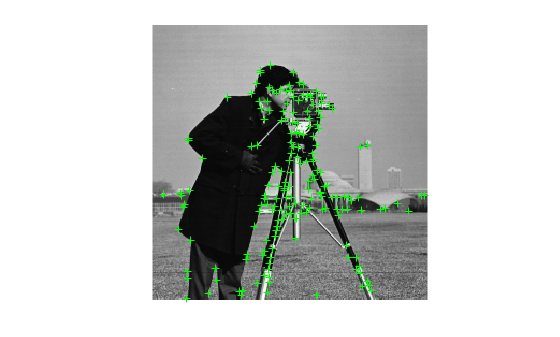# size

Return size of points object

## Syntax

``size(points)``
``sz = size(points)``
``sz = size(points,1)``
``sz = size(points,dimension)``
``[M,N] = size(points)``

## Description

example

````size(points)` returns the size of the points object.```
````sz = size(points)` returns the vector [`length`(`points`), 1].```
````sz = size(points,1)` returns the length of `points`.```
````sz = size(points,dimension)` returns the length of the dimension.```
````[M,N] = size(points)` returns `length`(`points`) for M and 1 for N```

## Examples

collapse all

`I = imread('cameraman.tif');`

Detect corner features.

`featurePoints = detectHarrisFeatures(I);`

Find the size of the feature points object.

`sz = size(featurePoints)`
```sz = 1×2 184 1 ```

Plot feature image with detected features.

```imshow(I); hold on; plot(featurePoints);```## Input Arguments

collapse all

Points object, specified as a `points` object. The object contains information about the feature points detected in the input image. To obtain points, use the appropriate detect function.

Dimension, returned as an integer. For `dim` >= 2, the object returns `1`.